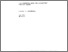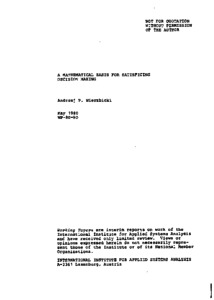# A Mathematical Basis for Satisficing Decision Making

Wierzbicki, A.P. (1980). A Mathematical Basis for Satisficing Decision Making. IIASA Working Paper. IIASA, Laxenburg, Austria: WP-80-090Preview
Text
WP-80-090.pdf

## Abstract

This paper presents an analysis of the satisficing decisionmaking process in a simple organization under multiple objectives. The role of aspiration levels or reference objective levels is stressed and a conceptual model of this behavior is presented.

A specification or rather modification of the mathematical concept of a value (utility) function that describes the satisficing behavior is given; the modified value function, called the achievement scalarizing function, should not be only order preserving but also order approximating in a certain sense. It is shown that the notions of reference objective levels and achievement scalarizing functions form a mathematical basis not only for satisficing decision making but also for Pareto optimization; this basis is an alternative to or even stronger than the approaches based on weighting coefficients or typical value functions. This mathematical basis, which can also be considered as a generalization of the goal programming approach in multiobjective optimization, results in pragmatic approaches to many problems of multiobjective analysis, including the problem of interactive assessment of solutions to economic models for policy analysis and planning purposes.

Item Type: Monograph (IIASA Working Paper) System and Decision Sciences - Core (SDS) IIASA Import 15 Jan 2016 01:48 27 Aug 2021 17:09 http://pure.iiasa.ac.at/1379View Item